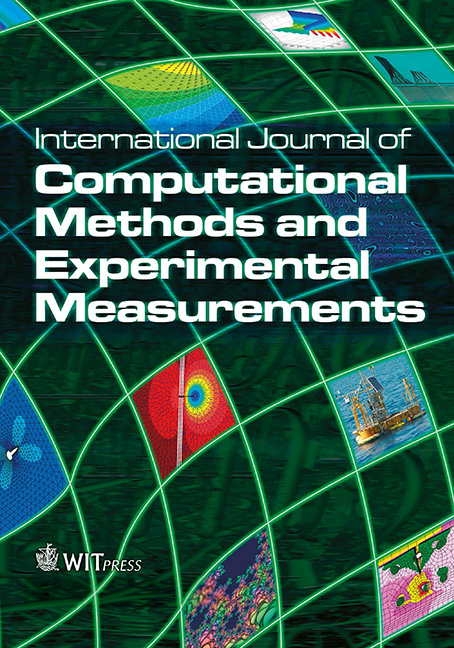WIT Press

BEM analysis of gravitational–capillarity waves on free surfaces of compound shells of revolution

Price

Free (open access)Volume

Volume 9 (2021), Issue 1

Pages

12

Page Range

38 - 50

Paper DOI

10.2495/CMEM-V9-N1-38-50

WIT Press

Author(s)

Vasyl I. Gnitko, Artem O. Karaiev, Maria L. Myronenko & Elena A. Strelnikova

Abstract

The paper presents a problem of gravitational–capillarity wave propagation in the frame of boundary integral equations. The wave propagation is considered in rigid compound shells of revolution. The liquid is supposed to be an ideal and incompressible one, and its flow is irrotational. The boundary value problem is formulated for Laplace’s equation to obtain the velocity potential. Non-penetration boundary conditions are used at the shell’s wetted surface, as well as kinematic and dynamic boundary conditions are given on the free liquid surface. Effects of surface tension are included in the Bernoulli’s equation as additional pressure that is proportional to the free surface mean curvature. It allows us to consider coupled effects of both gravitational and capillarity waves. The problem is reduced to a system of singular integral equations. For their numerical simulation, the boundary element method is in use. The singular integral equations in implementation of a discrete model are transformed to linear algebraic ones, and eigenvalue problems are solved for different capillarity length numbers. Benchmark numerical investigations are presented including different kinds of compound rigid shells.

Keywords

boundary element method, gravitational–capillarity wave, rigid compound shells, singular integral equations, surface tension.